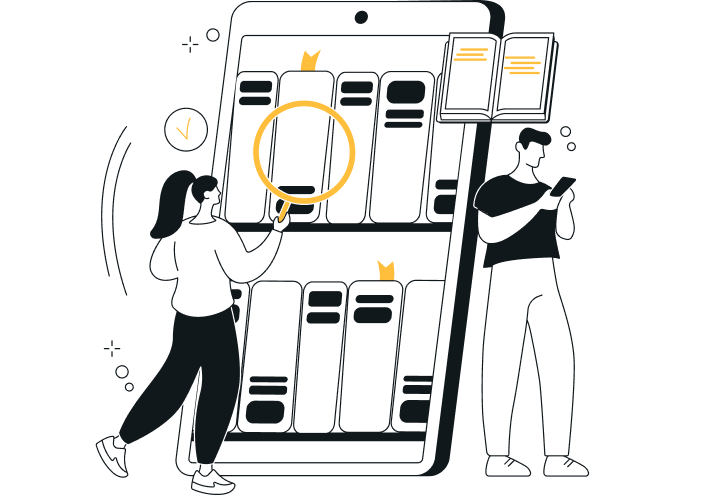# Rainbow products

Category: Product
Last Updated: 27 Aug 2020
Pages: 2 Views: 136

#### Rainbow Products

• A) Payback = 7 years; NPV = -\$945.68; IRR = 11.49. Rainbow should not purchase because the IRR is less than the cost of capital and also the NPV is negative.
• Order custom essay Rainbow products with free plagiarism report450+ experts on 30 subjectsStarting from 3 hours delivery
Get Essay Help
• B) PV of the Perpetuity = C/r = \$37,500; NPV = Investment + PV = \$2,500. Since this NPV is positive, Rainbow should purchase the service contract.
• C) V = C/(k-g) = \$50,000; NPV = \$15,000. Rainbow Products should accept this option due to its positive NPV. This would be the best choice for Rainbow Products since it has the largest NPV.

#### Hot Dog Stand

• A) Using the IRR, I would recommend the 1207.6% or the rent a larger stand option.
• B) Using the NPV, I would recommend the \$34,825.76 of building a new stand.
• C) IRR shows the return on the money spent while NPV shows the future dollar value of the investment. An IRR will look good if the initial investment is low but NPV shows a better picture of what can be earned back in dollar values. That is why it is better to follow the NPV rule over the IRR rule.

#### Lockheed Tri-Star

1. At the planned production level of 210 units at an estimated cost of \$14 million and piece the NPV was -\$584 million.
2. Even the industry analysts seemed to be wrong with their breakeven point of 300 units because the NPV at 300 units was -\$247 million. This was at a modest discount rate of 10% even though the cost of capital was probably higher due to the riskiness of the investment.
3. To predict the sales volume that would reach the true economic break-even for Tri-Star means that a couple of variables have to be estimated. Due to a learning curve, I have estimated the costs per unit and placed them in the table below. Using excel, I used a trial and error set up to work the number of needed production by getting NPV as close to zero as possible. My calculations brought me to 458 aircraft with a cost of \$11.5 million per aircraft.
4. This decision was a terrible idea and an extremely unreasonable decision. This was a case of over-ambition and poor analysis that lead a company in a bad direction. This bad project reduced its stock price to \$11 from the \$64 it was 4 years prior.